# High school geometry questions and answers

Recent questions in Geometryf480forever2rz 2021-11-29 Answered

### An inverted cone has a height of 15 mm and a radius of 16mm. The volume of the inverted cone is decreasing a rate of 534 cubic mm per second, with the height begin held constant. What is the rate of change of the radius, in mm per second, when the radius is 6 mm? Round your answer to the nearest hundreth. (Do not include any units in your answer Remember that the volume of a cone is $$\displaystyle={V}=\frac{{1}}{{3}}\pi{r}^{{{2}}}{h}$$veudeje 2021-11-29 Answered

### Solve each of the following triangles. If there are two triangles, solve both. Round angle measurements to one decimal place and side lengths to two decimal places. $$\displaystyle{a}={9},{b}={4},{B}={23}^{{\circ}}$$ a= y= c=folklorahhe 2021-11-29 Answered

### Triangles ABC and DEF are right triangles, as shown. $$\displaystyle\triangle{A}{B}{C}$$ is similar to $$\displaystyle\triangle{D}{E}{F}$$. Which ratios are equal to $$\displaystyle{\cos{{\left({B}\right)}}}$$ ? Choose the TWO ratios that apply.Tomasettiq3 2021-11-29 Answered

### What is the volume of this cone? Use $$\displaystyle\pi\approx{3.14}$$ and round your answer to the nearest hundredth. What is the volume of this cylinder? Use $$\displaystyle\pi\approx{3.14}$$ and round your answer to the nearest hundredth.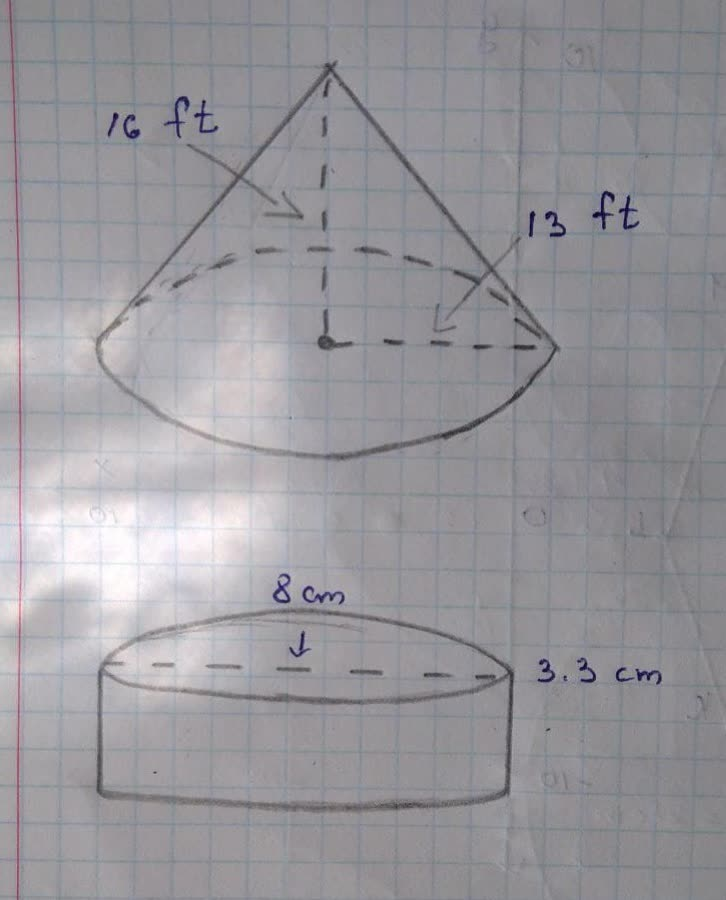jippie771h 2021-11-29 Answered

### What is the circumference of the circle? Use $$\displaystyle{\frac{{{22}}}{{{7}}}}$$ for x. a) 44 cm b) 66 cm c) 182 cm d) 154 cmEdmund Adams 2021-11-28 Answered

### A pole has two wires attached to it, one on each side, forming two right triangles as shown.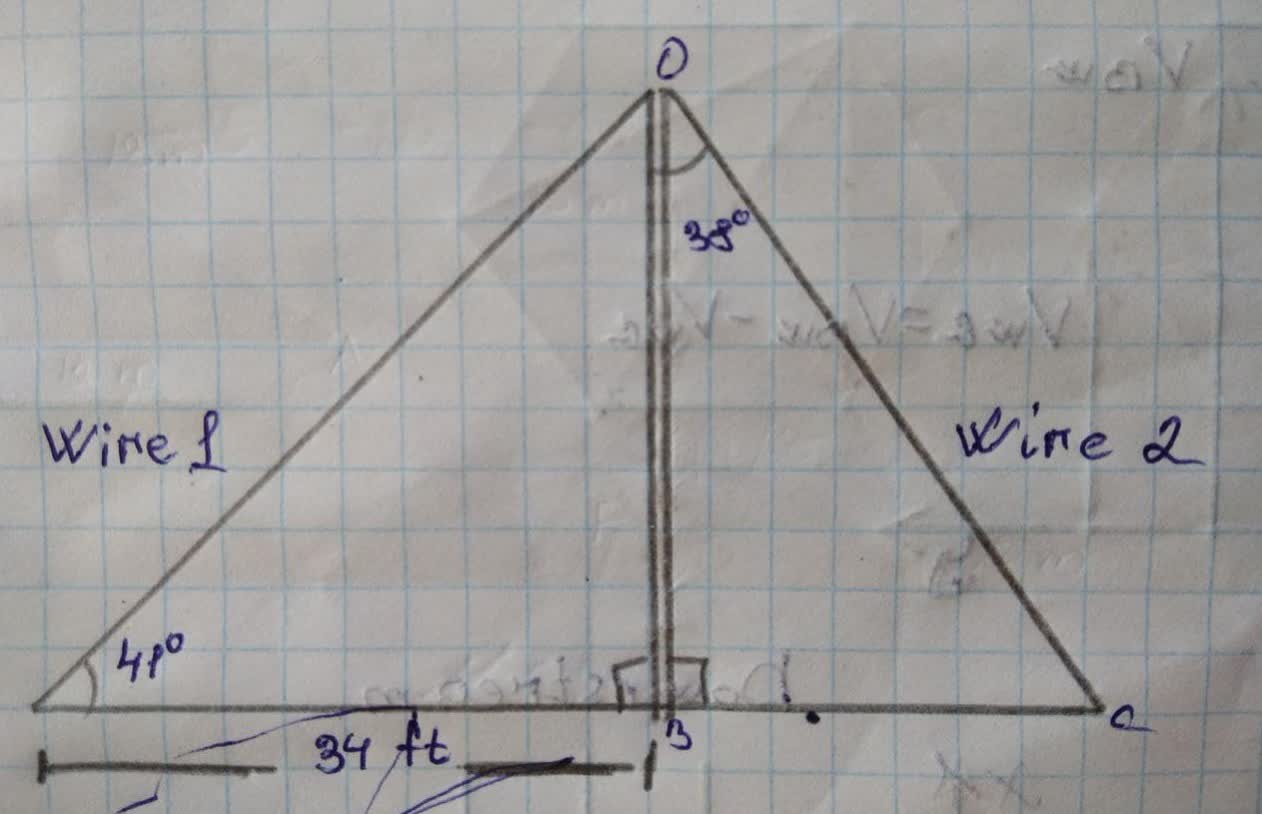ostric16 2021-11-28 Answered

### Triangles ABC and DEF are similar triangles. Use this fact to solve the exercise. Round to the nearest tenth. Find the perimeter of triangle ABC.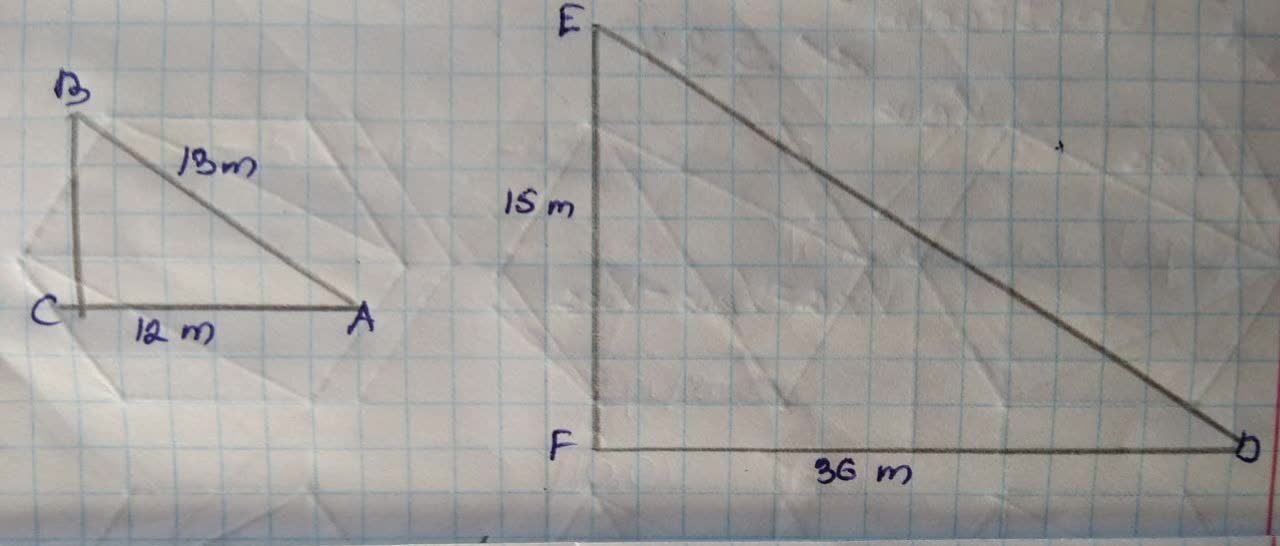Guenuegoomyns 2021-11-28 Answered

### What is the Volume of this sphere? Use $$\displaystyle\pi\approx{3.14}$$ and round your answer to the nearest hundredth, Maple syrup in a bottle has a mass of 918 grams and a density of 1.36 grams per cubic centimeter. What is its volume?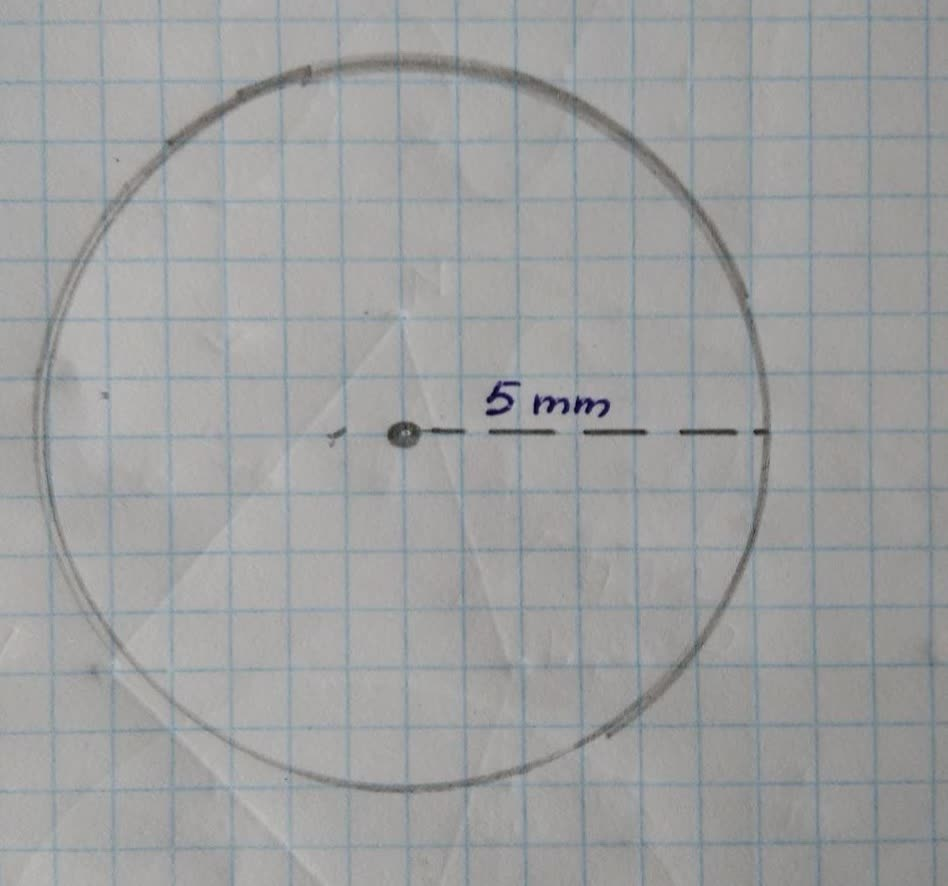Jason Watson 2021-11-28 Answered

### What is the value of t?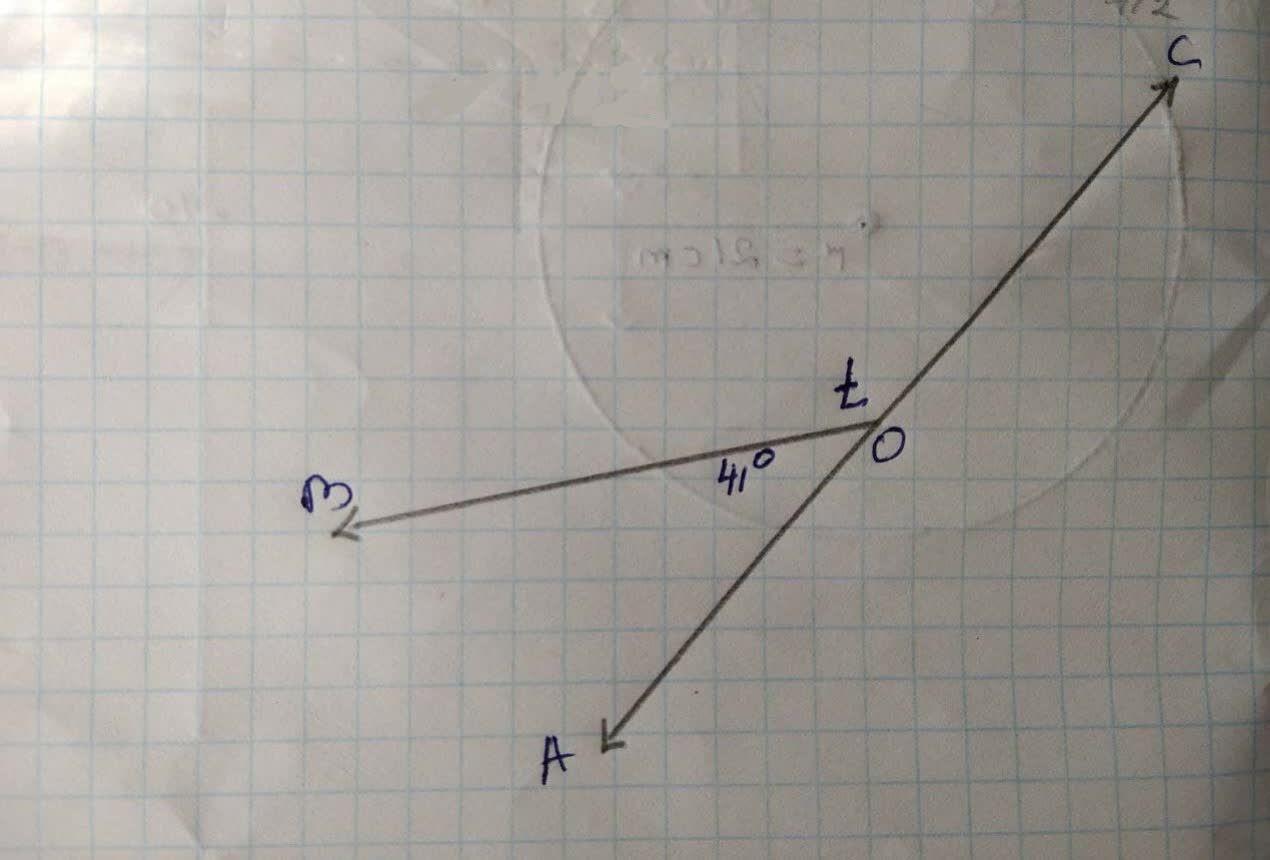jeseHainsij 2021-11-28 Answered

### Analytic Geometry: Trace the curve $$\displaystyle{y}^{{{2}}}={\left({x}−{1}\right)}{\left({2}{x}+{3}\right)}{\left({3}{x}−{1}\right)}.$$Ashley Searcy 2021-11-27 Answered

### triangle ABC is a right triangle with its right angle at C. The bisector of angle B intersects AC at D. The bisector of the exterior angle at B intersects AC at E. If BD= 15 and BE=20, what are the lengths of triangle ABC?TokNeekCepTdh 2021-11-27 Answered

### Use the Law of Cosines to solve the triangles. Round lengths to the nearest tenth and angle measures to the nearest degree. $$\displaystyle{a}={4},\ {b}={6},\ {c}={9}$$ $$\displaystyle{a}={4},\ {b}={7},\ {c}={6}$$Douglas Kraatz 2021-11-27 Answered

### Analytic Geometry: Trace the curve of $$\displaystyle{x}^{{{3}}}-{6}{x}^{{{2}}}+{11}{x}-{y}={6}$$Lloyd Allen 2021-11-26 Answered

### Use the Law of Cosines to solve the triangles. Round lengths to the nearest tenth and angle measures to the nearest degree. PLEASE solve both of these triangles. Thank you!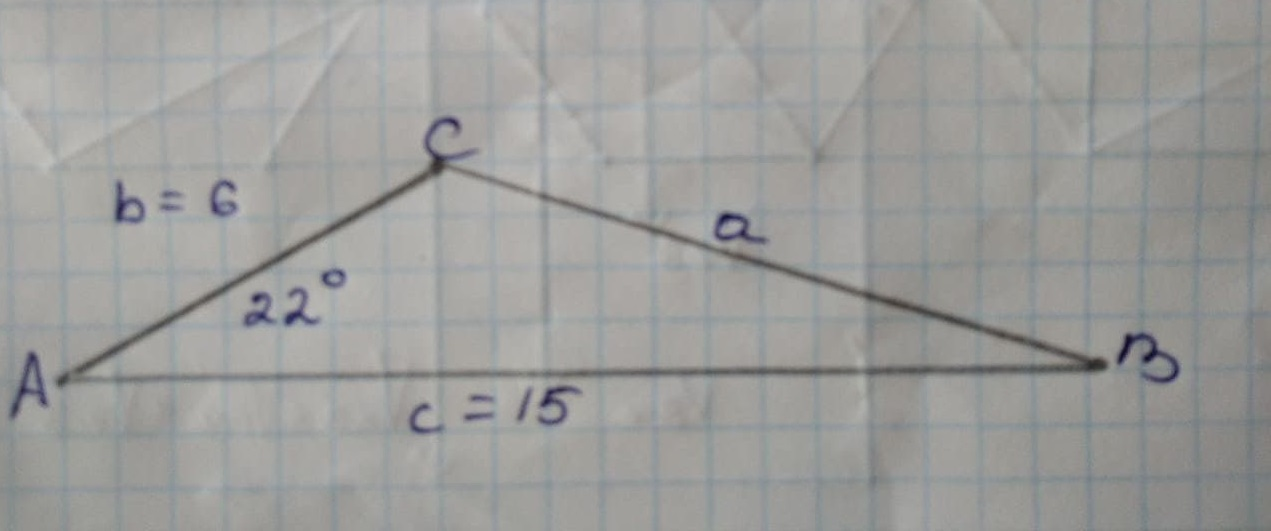lenlifiauw2 2021-11-26 Answered

### Use the Law of Cosines to solve the triangles. Round lengths to the nearest tenth and angle measures to the nearest degree. $$\displaystyle{a}={5},\ {b}={5},\ {c}={5}$$ $$\displaystyle{a}={66},\ {b}={25},\ {c}={45}$$Tomasettiq3 2021-11-26 Answered

### Using analytic geometry, find the coordinates of the point on the X axis which is equidistant from A ( 5,8 ) and B(−3,4).Sheelmgal1p 2021-11-26 Answered

### What is EG?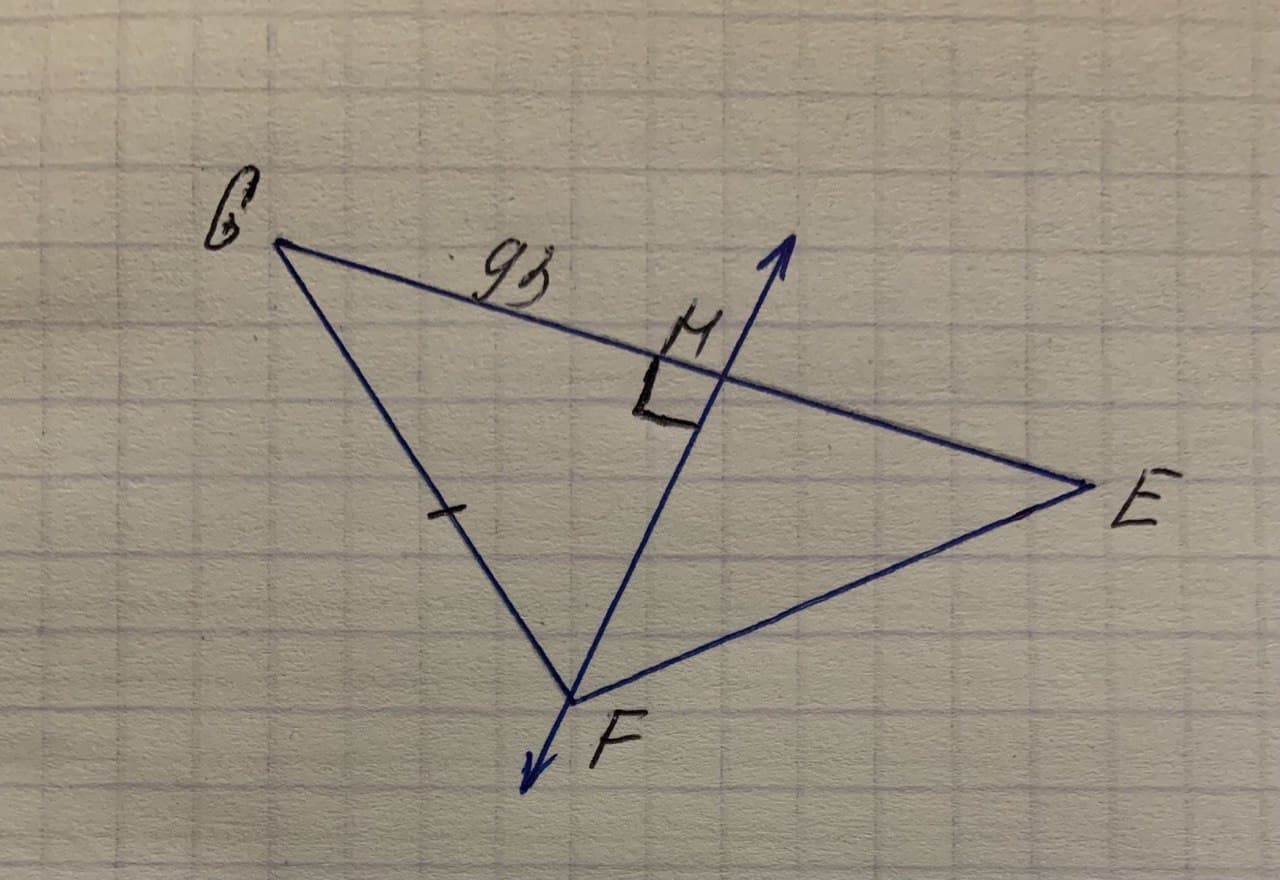Carolyn Moore 2021-11-25 Answered

### Solve each of the following triangles. If there are two triangles, solve both. Round angle measurements to one decimal place and side lengths to two decimal places. $$\displaystyle{a}={10},\ {b}={12},\ {c}={7}$$ a=? B=? y=?pamangking8 2021-11-25 Answered

### Given $$\displaystyle{b}={2},\ {a}={3}$$, and $$\displaystyle{B}={40}$$ (degrees), determine whether this information results in one triangle, two triangles, or no triangle at all. Solve any resulting triangles.podnescijy 2021-11-25 Answered

### The perpendicular bisector $$\displaystyle\overline{{{A}{B}}}$$ in the right triangle $$\displaystyle\triangle{A}{B}{C}$$ forms the triangle with the area 3 times smaller than the area of $$\displaystyle\triangle{A}{B}{C}$$. Find the measures of acute angles in $$\displaystyle\triangle{A}{B}{C}$$

Unfortunately, Geometry does not receive sufficient attention from high school students because it is not taught with due explanations. Knowing it well, we provide high school students and parents with high school Geometry questions and answers to make things easier for you as you have to cope with yet another task.

It is not like the list of answers at the end of your textbook because we also have practice answers and various questions answered where you can find the most efficient solutions and see certain rules that make Geometry feel much easier!

...